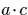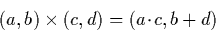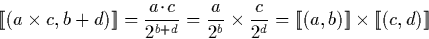Next: Shift operators Up: Dyadic Digit Operations Previous: Average

# Multiplication

Multiplication of dyadic digits is simpler than the average operation. Given two dyadics (a,b) and (c,d), we know that a and c are both odd or zero. Hence, if either a or c are zero, the result will be the dyadic digit (0,0). Otherwise,is odd, so the result is:becauseMartin Escardo
5/11/2000